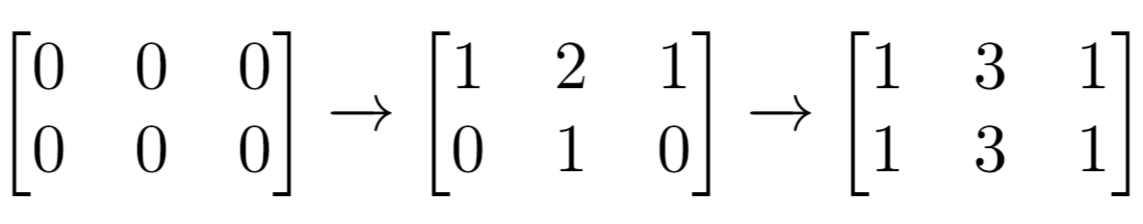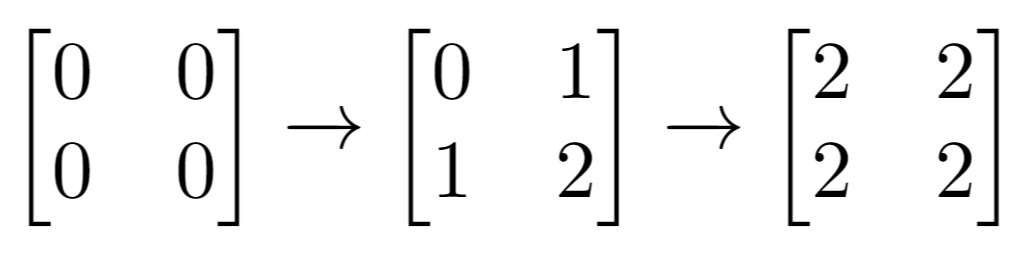1252. Cells with Odd Values in a Matrix

#### QUESTION:

Given n and m which are the dimensions of a matrix initialized by zeros and given an array indices where indices[i] = [ri, ci]. For each pair of [ri, ci] you have to increment all cells in row ri and column ci by 1.

Return the number of cells with odd values in the matrix after applying the increment to all indices.

Example 1:Input: n = 2, m = 3, indices = [[0,1],[1,1]] Output: 6 Explanation: Initial matrix = [[0,0,0],[0,0,0]]. After applying first increment it becomes [[1,2,1],[0,1,0]]. The final matrix will be [[1,3,1],[1,3,1]] which contains 6 odd numbers. Example 2:Input: n = 2, m = 2, indices = [[1,1],[0,0]] Output: 0 Explanation: Final matrix = [[2,2],[2,2]]. There is no odd number in the final matrix.

Constraints:

1 <= n <= 50 1 <= m <= 50 1 <= indices.length <= 100 0 <= indices[i] < n 0 <= indices[i] < m

#### EXPLANATION:

1.首先对二维数组进行变化
2.将变化结果进行计算，查看是否是奇数偶数

#### SOLUTION:

``````class Solution {
public int oddCells(int n, int m, int[][] indices) {
int[][] array = new int[n][m];
for(int i = 0;i<indices.length;i++){
int row = indices[i];
oddCellsHelper(array,row,-1);
int col = indices[i];
oddCellsHelper(array,-1,col);
}
int result = 0;
for(int i = 0;i<array.length;i++){
for(int j = 0;j<array[i].length;j++){
if(array[i][j] %2 ==1) result++;
}
}
return result;
}
public static void oddCellsHelper(int[][] arr,int row,int col){
if(row!=-1){
for(int i = 0;i<arr.length;i++)
arr[row][i]++;
}else{
for(int i = 0;i<arr.length;i++)
arr[i][col]++;
}
}
}
``````